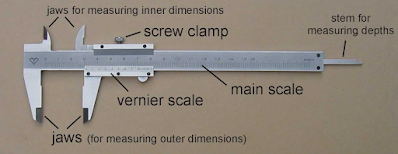Vernier Caliper: Least count, Least count of Vernier Caliper ~ MECHTECH GURU

# Vernier Caliper

It is a visual aid that allows the user to measure more precisely than could be done unaided when reading a uniformly divided straight or circular measurement scale.

## Least count

The least count of a measuring instrument is the smallest change in the measured quantity that can be resolved on the instrument's scale.Vernier Caliper

## Least count of Vernier

It is the difference between the value of one Main scale division and the value of one Vernier scale division.

Let the smallest main scale reading, that is the distance between two consecutive graduations (also called its pitch) be S and the distance between two consecutive Vernier scale graduations be V such that the length of (n-1) main scale divisions is equal to n Vernier scale divisions.

Then, the length of (n-1) main scale divisions = the length of n vernier scale division

or, (n-1)S=nV

or, nS-S=nV

or, S/n = S -V

or (Pitch)/(Number of Vernier scale divisions) = (Length of one

main scale division - Length of one Vernier scale division)

So, S/n and (S - V) are both equal to the least count of vernier scale.

## Example of Least count of  Vernier Caliper

Ten divisions on the vernier scale coincide with 9 smallest divisions on the main scale (mm), Main scale Reading is 2.6 cm and vernier scale coincides with 7 division of the main scale.

a) Calculate the Least Count(L.C.) of the vernier scale.

Solution:

L.C. = Value of one main scale division - Value of one vernier scale division

L.C. = 1 mm- 9/10mm = 0.1mm = 0.01cm